﻿ Tilak De Silva - Profession

## Summery of Logic Gates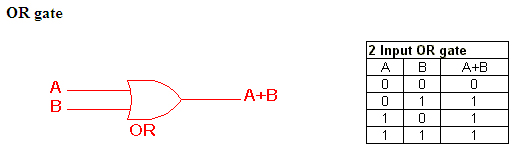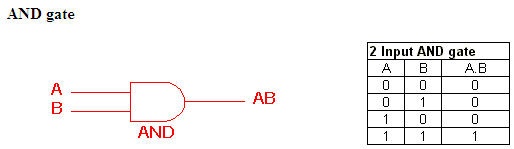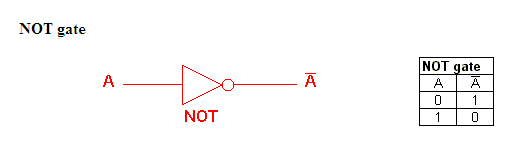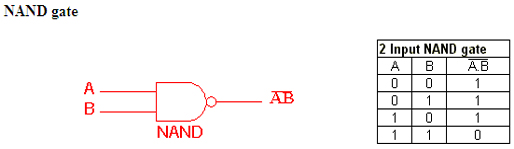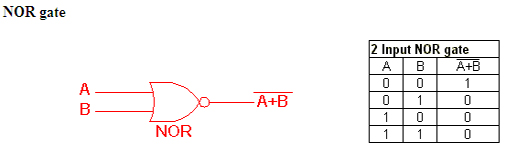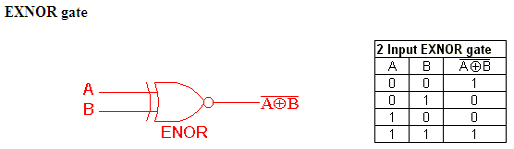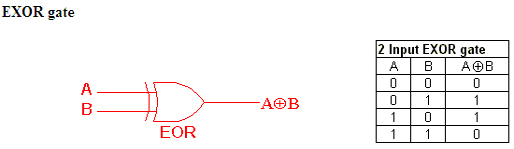The (axioms) rules of Boolean Algebra are:
 AND Operations (•) 0·0 = 0     A·0 = 0 1·0 = 0     A·1 = A 0·1 = 0     A·A = A 1·1 = 1     A·A' = 0 OR Operations (+) 0+0 = 0     A+0 = A 1+0 = 1     A+1 = 1 0+1 = 1     A+A = A 1+1 = 1     A+A' = 1 NOT Operations ( ' ) 0' = 1     A' = A 1' = 0

 Commutative Law Associative Law A·B = B·A A+B = B+A (A·B)·C = A·(B·C) = A·B·C (A+B)+C = A+(B+C) = A+B+C Distributive Law Idempotent Law A·(B+C) = (A·B) + (A·C) A+(B·C) = (A+B) · (A+C) A·A = A A+A = A

DeMorgan’s Theorem
(A·B)' = A' + B' (NAND)
Not A and B is combined operator which is called NAND
(A+B)' = A'·B' (NOR)
Not A or B is combined operator which is called NOR

Virtual Labs - Logic Gates
(Resources from Internet)